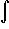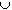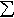#Interactive Real Analysis

Next | Previous | Glossary | Map

## 7.4. Lebesgue Integral

### Example 7.4.11(d): Properties of the Lebesgue Integral

Suppose f is a bounded, non-negative function defined on a measurable set E with finite measure such thatE f(x) dx = 0. Show that f must then be equal to zero except on a set of measure zero.
Define the sets
En = { xE: f(x)1/n }
Z = { xE: f(x) # 0 }
ThenEn = Z and EnE. Using the previous two examples we get:
0 =E f(x) dxEn f(x) dx1/n m(En)
so that m(En) = 0 for all n. But then
m(Z) = m(En)En = 0
which is what we had to prove.
Next | Previous | Glossary | Map